# Mathway (lite)

## Review Overview

### 6.3

Summary : Supporting you in understanding and solving almost every type of math problem!

[ios_app id=”467329677″]

Mathway allows users to input a variety of math problems—ranging from basic math through advanced statistics—and it automatically solves each problem. If users pay for the upgrade, Mathway will also explain the steps it used to solve the problem. Additionally, Mathway contains a large library of example problems users can view, and a glossary of mathematical terms.

### Instructional Ideas

1. When teaching about a specific type of math problem, teachers can have students view the example problem in this app. Next, students would then create an additional math problem on their own that is the same type of math problem and solve it.
2. Teachers can assign students specific math terms that they must look up using this app’s glossary, and then create an illustration to exemplify the term. For example, if assigned the term “acute angle,” a student would have to draw an angle that is less than 90 degrees.
3. Students can solve math problems independently and then check their work using this app.
4. When viewing an example problem and its solution, teachers can have students write an explanation for how this app solved the problem. By composing these explanations, students will develop their conceptual understanding for a variety of mathematical concepts.
 A1. Rigor A2. 21st Century Skills A3. Conn. to Future Learning A4. Value of Errors A5. Feedback to Teacher A6. Level of Material A7. Cooperative Learning A8. Accom. of Individual Diff.
 B1. Ability to Save Progress B2. Platform Integration B3. Screen Design B4. Ease of Use B5. Navigation B6. Goal Orientation B7. Information Presentation B8. Media Integration B9. Cultural Sensitivity
 C1. Learner Control C2. Interactivity C3. Pace C4. Flexibility C5. Interest C6. Aesthetics C7. Utility

### Screenshots

•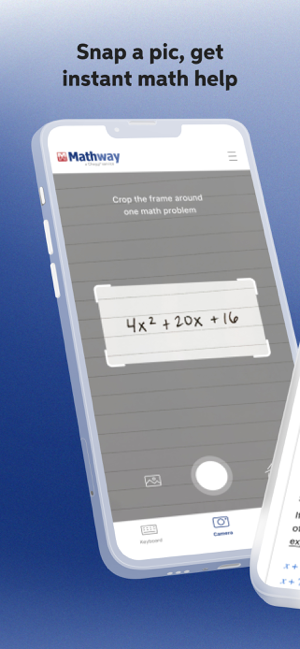•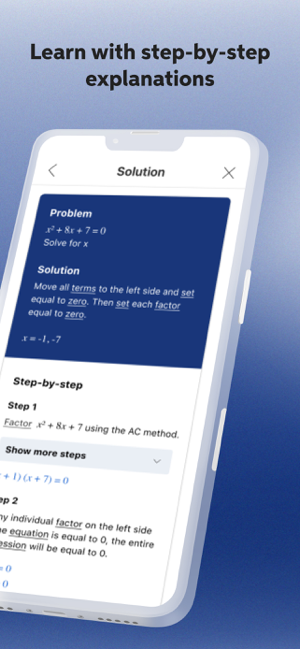•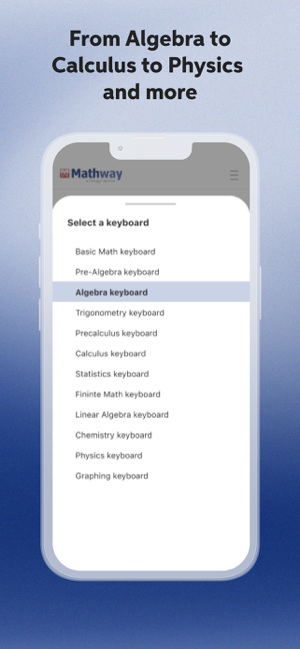•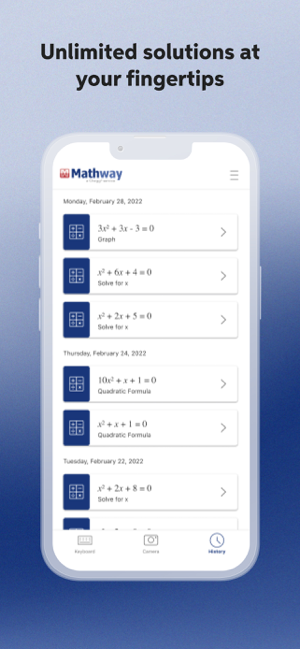•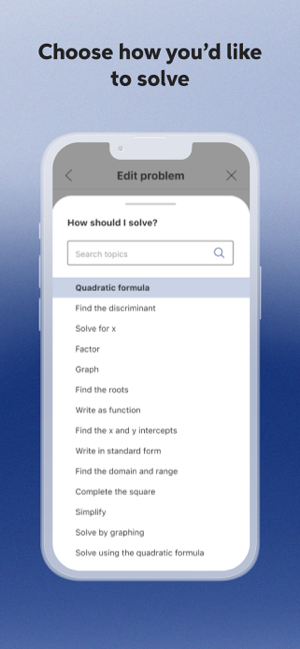•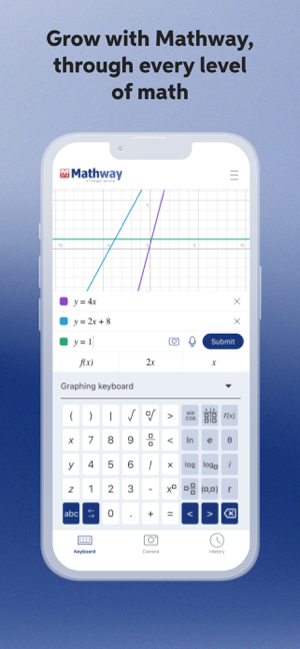•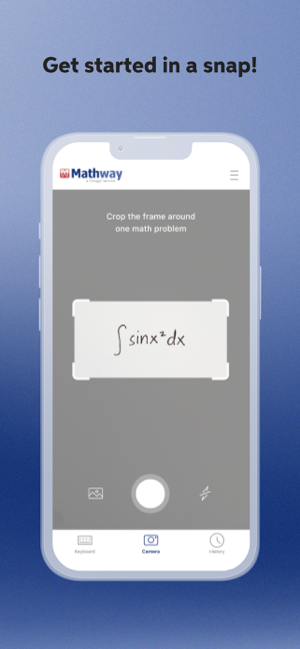•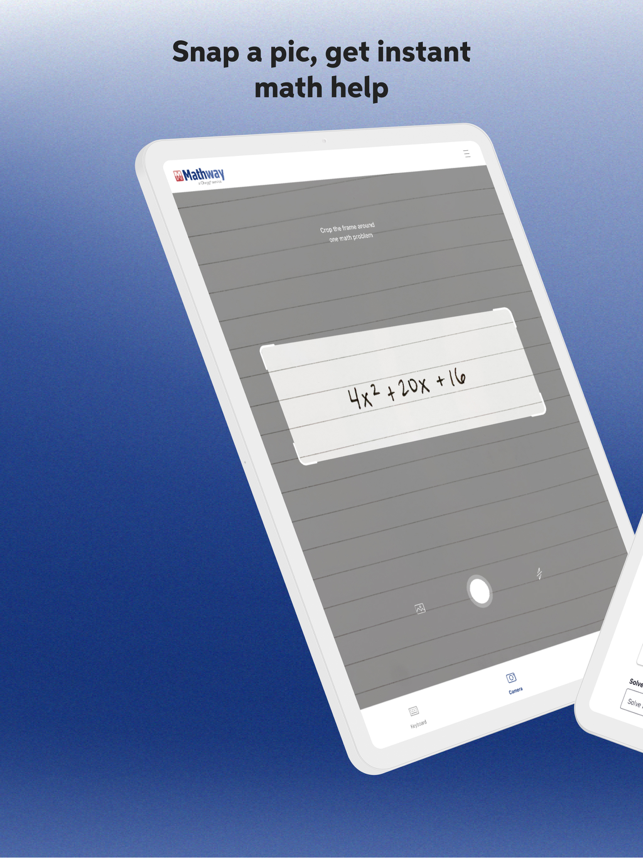•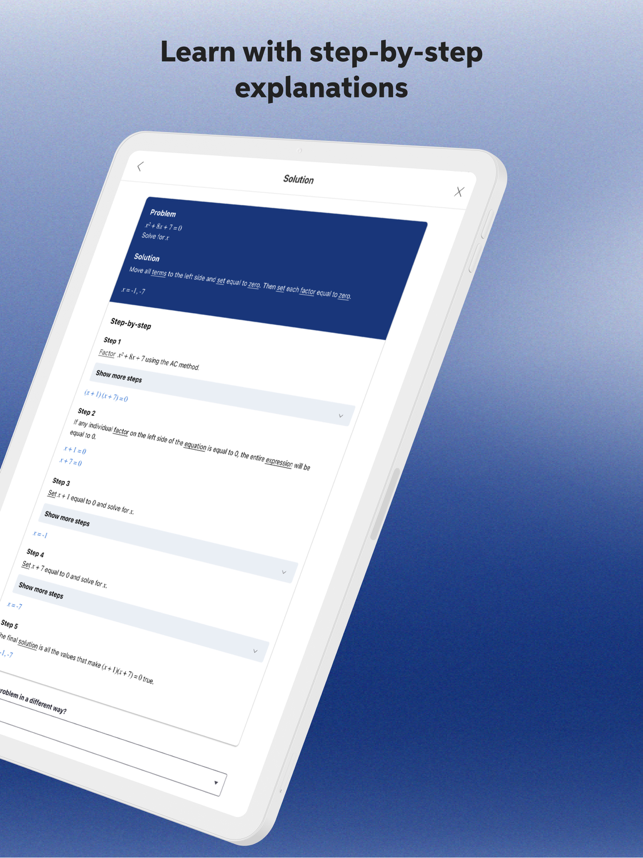••••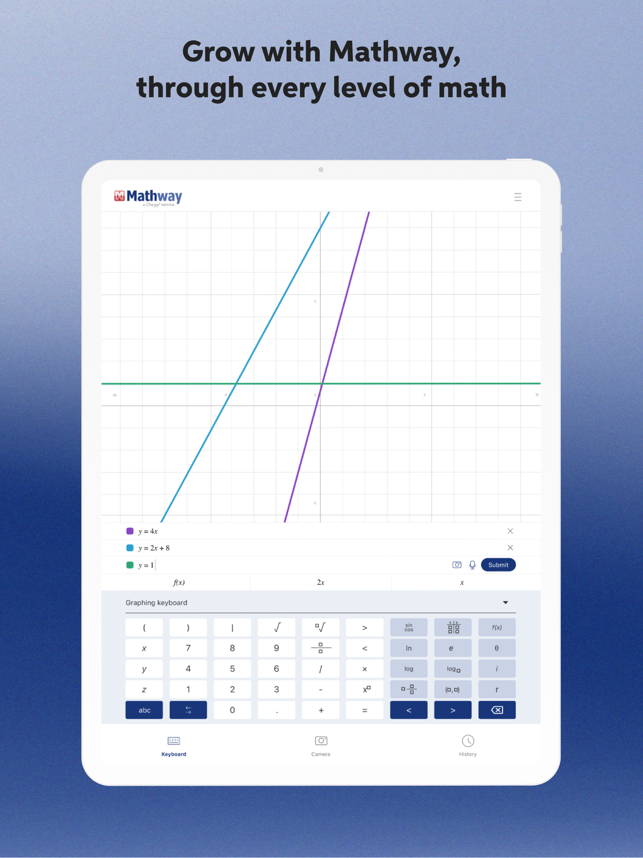•